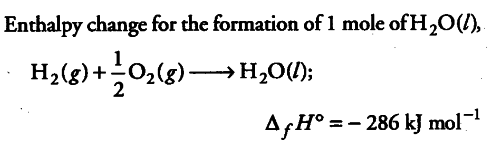# Calculate the entropy change in surroundings

Calculate the entropy change in surroundings when 1.0 mole of \$H _{ 2 }O\$(l) is formed under standard conditions. ∆fH° = -286 kJ/molEnergy released in the above reaction, is absorbed by the surroundings.∆S ={ q }_{ surr } / T
= +286 kJ/mol / 298K
= 0.9597 kJ /K/mol
= 959.7 Jk/Mol